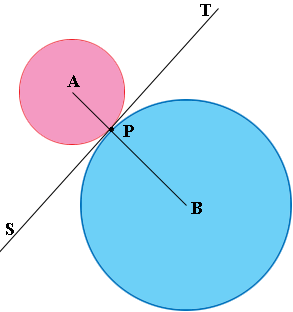SEARCH HOMEMath Central Quandaries & QueriesQuestion from mark, a student: Three circles of radii 24 cm, 32 cm, and 42 cm are externally tangent to each other (each is tangent to the other two). Draw a diagram and using the Law of Cosines find the largest angle of the triangle formed by joining their centres.Hi Mark,

I drew two circles with centres $A$ and $B$ that are tangent to each other at the point $P.$I joined $A$ and $B$ to $P$ and drew the common tangent line $ST.$ Since $AP$ is a radius of the small circle and $PT$ is tangent to the circle, the angle $APT$ is a right angle. Similarly the angle $TPB$ is a right angle. Thus $AB$ is a straight line.

This is the key to your problem. See if you an solve the problem and write back if you need more assistance.

PennyMath Central is supported by the University of Regina and The Pacific Institute for the Mathematical Sciences.